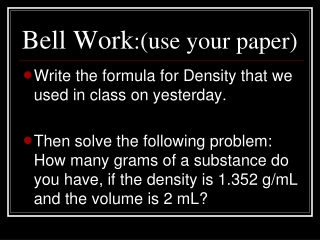DownloadDownload PresentationBell Work :(use your paper)

# Bell Work :(use your paper)

Download Presentation## Bell Work :(use your paper)

- - - - - - - - - - - - - - - - - - - - - - - - - - - E N D - - - - - - - - - - - - - - - - - - - - - - - - - - -
##### Presentation Transcript

1. Bell Work:(use your paper) • Write the formula for Density that we used in class on yesterday. • Then solve the following problem: How many grams of a substance do you have, if the density is 1.352 g/mL and the volume is 2 mL?

2. Page 15 of Scientific Notebook Density What is it………….

3. Objectives • Evaluate Density in terms of mass and volume SI Units. • Use the Guess Method to solve problems involving density, mass, and volume.

4. SPi’s & CLE’s: • CLE 3221.Inq.1 Recognize that science is a progressive endeavor that reevaluates and extends what is already accepted. • SSPI 3221.Math.2 Perform operations on algebraic expressions and informally justify the selected procedures. PI 3221.Math.2 Perform operations on algebraic expressions and informally justify

5. What is Density? • The ratio of mass to volume • Physical property • Can be used to identify substances

6. Why is density important? • Every substance has a particular density. (Why is this Important?)

7. Answer: • Density can be used to identify unknown substances. • Density can be used to determine if a substance is pure.

8. Calculations & Density • Density is calculated as follows: Density = Mass Volume Or D = m V

9. Page 16 of Scientific Notebook Problem Solving and Density Manipulate the density formula and solve for Mass, Volume.

10. What SI Unit does density have? • Density = ? • Mass = grams • Volume = mL or cm3 • Density = g/mL or g/cm3

11. Problem Solving: Calculate the density of a block of lead with dimensions 20 cm x 8.0 cm x 35 mm, has a mass of 6356 g.

12. Problem Solving: • What is the volume of 227 g of olive oil if its density is 0.92 g/mL? • Calculate the mass of a substance if its volume is 95.7mL and its density is 1.58g/mL.

13. Enrichment: • Ocean Currents – circulation in the ocean is density driven by differences in temperature and salinity. The thermohaline circulation in the oceans is responsible for ocean currents. Surface winds push water at the surface toward the poles from the equator. As the warmer surface water moves toward the poles, it cools and becomes denser. Evaporation causes the salinity of water to become greater. This water will sink because it becomes denser.

14. Density Logic/Group Collaboration • Please form groups of three • Sit quietly for further instructions

15. Density Logic Puzzle

16. Closure: • Write two things that density can be used for…….(T-O-T-D).

17. Homework: What is superposition? How is superposition related to Density?